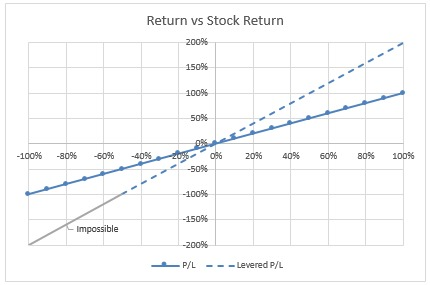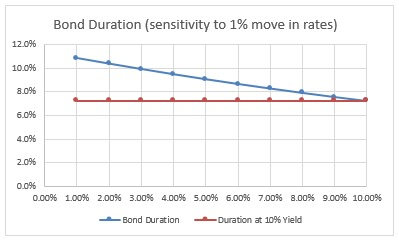# Where Does Convexity Come From?

Unless you are a bond or derivatives trader, the term “convexity” usually just makes you want to say “beat it, nerd”.  As if to frustrate us further it has many aliases: “curvature”, “non-linearity”, “gamma”.  It turns out, for such a fancy word “convexity” is quite approachable.

In this post, we will learn:

• what it looks like
• common places to find it
• why you should care

But first, let’s see what convexity isn’t.

### Linear P/L

If you buy 1 share of stock for \$50 and it goes up 5% you make \$2.50. You make 5%. If it goes up 10% you make 10%. Your P/L is linear with respect to what the stock does.Leverage does not change the fact that owning a stock has a linear payoff. Leverage may amplify the volatility of the position but not the linearity.  In the chart below, the Levered P/L assumes you buy the same stock but use 50% margin (you borrow \$25 out of the \$50 it took to buy the stock). So if the stock returns 20% (\$50 –> \$60), you return 40% (\$10 profit on a \$25 outlay). In other words, the slope of your P/L with respect to the stock is 2x the unlevered return.Futures, since they are traded on margin, are levered. But like stocks, they have a linear payoff.  How about a house? If you buy a house with 20% down and it appreciates 20%, you will make 100% on your money. If it appreciates 40% you will make 200%. Your P/L slope is 5x because of the leverage but most importantly it is constant. The rise/run to give you geometry flashbacks is always 5. You can change the slope by paying down the mortgage but changes in the slope are not an intrinsic feature of the investment. Most assets we are used to buying or selling have linear payoffs.

Leverage increases the volatility of the position but leverage does not mean convexity.

### Exposures As Deltas

The term “delta” is familiar to many as the option greek which represents a hedge ratio. It answers the question, how much will my option value change per \$1 change in stock. I can use that ratio to compute how much stock I would need for an equivalent position.

100 50% delta calls is equivalent to 5,000 shares of stock (100 x .50 delta x 100 share multiplier per option). If I were bullish, I could buy the stock and say I’m long 5,000 deltas. I could also have chosen to buy 100 50% delta calls. I would still say I’m long 5,000 deltas. This allows me to collapse either exposure into the statement “if the stock goes up \$1, I will make \$5,000”.

We are now using the word “delta” to refer to an exposure. We are agnostic as to whether it’s stock or call options. Either way, we have the same root on — we want the stock to go up, and we know how much we will make when it does.

In the stock example, every dollar change led to a constant P/L change. This is because your exposure is staying constant. We can refer to your exposure as your delta. Instead of referring to the sensitivity of an option to its stock, we are using delta as the slope of your P/L with respect to the stock. This allows us to generalize any exposure into a slope.

Example of a typical call overwriter:

• You own 3,000 shares of stock = long 3,000 deltas.
• You are short 30 20% delta calls (equivalent of 600 shares) = short 600 deltas

Net delta: Long 2,400.

This represents the slope of your P/L with respect to the stock. If the stock goes up \$1, you make \$2,400. If the stock goes down \$1, you lose \$2,400

In fact, this is how pros combine their positions. They want to know their aggregate delta, or exposure due to changes in the stock price. In fact, pros may even use the correlation between stocks to estimate a net delta with respect to say the SP500. A fund that is trying to stay market neutral might have a basket of alpha-generating longs hedged with a delta equivalent amount of short index futures.

A typical end-of-day report might include the firm’s best estimate of delta with respect to the Nasdaq, delta with respect to SP500, delta with respect to Eurostoxx, and so forth.

It’s worth restating:

Think of a position as a “delta” — the slope of your P/L to a market.

#### Not All Deltas Are Created Equal

Let’s compare the deltas of a long stock position vs a long call position. Both positions are long 5,000 deltas

• Stock position

Instrument: 5,000 shares
P/L: \$5,000 per \$1 change in stock

• Call Position

Instrument: 100 50% delta calls
P/L: \$5,000 for the first \$1 change in stock

Bang! Imagine the stock went to \$100. At \$100, the stock position is still making \$5,000 per \$1 change. But the call position is now making \$10,000 per \$1 change.

Why?

Because the 50 strike is so deep in-in-the-money that the calls now have 100% delta. The calls are now equivalent to a 10,000 share position! (100 calls x 1.00 delta x 100 share multiplier). The call owner is long 10,000 deltas now. At \$100, your delta per \$1 change is \$10,000 not \$5,000.

Here’s the delta of that 1 year 50 strike call at different stock prices.Contrast this with the stock owner, who still has just 5,000 deltas. Pure stock is a linear exposure. Linear instruments have constant slopes with respect to the market. They are benign. If you are long 5,000 stock deltas you don’t suddenly find yourself long 10,000 deltas. Stock and futures can be said to be 100% delta instruments. The P/L moves 1 for 1 with the asset price.

But options payoff are non-linear because your delta, your exposure, is actually changing. This is the key to identifying convexity. Payoffs are non-linear not because the asset is volatile. Payoffs are non-linear because the delta of your position is changing. This in turn causes the slope of your P/L with respect to the market’s moves to change.

If you are dealing with an instrument whose exposure changes for the same given change, you are dealing with a non-linear exposure.

### Curved P/L

Curvature is a nice term because it reminds us that we are concerned with the shape of a payoff. Curvature indicates that the slope of your payoff changes. Your delta is morphing due to time, changes in volatility, and in these examples, due to changes in the asset price.

If the slope of the payoff is changing then our P/L will curve with respect to changes to a variable or asset price.

#### Options

Earlier we saw that stock P/L’s are constant. They have a constant slope because their deltas don’t change. Your P/L matches the stock’s changes. It’s just weighted by the size of your position.Option deltas do change. We know a call delta varies between 0 and 100%. Imagine a stock is trading for \$40. You buy a 1 year 50 strike call @ 16% vol. It will start with a 9% delta. Curvature refers to the idea that the delta of that call does not stay constant. As the stock rallies, your delta grows allowing you to make much more than \$.09 per \$1 change.When we zoom into the classic hockey stick graph of a call option value with respect to stock we can see the curvature. It’s the difference between assuming a constant \$.09 per dollar and what actually happens as the option gains sensitivity to the stock price.

If you drive a car 30mph for 30 minutes you will travel 15 miles. If 10 minutes into the trip you instantly accelerated to 60mph and stayed at the speed for 20 more minutes you will find that you have traveled 25 miles. The difference between 25 miles and your original linear estimate of 15 miles is curvature.

In the analogy, your velocity at any one point in time is your delta. The change in velocity as you went up a gear was a change in delta (and Greekophiles will recognize the acceleration as gamma).

If you want the opportunity to test your understanding go back to the call overwriter example:

• You own 3,000 shares of stock = long 3,000 deltas.
• You are short 30 20% delta calls (equivalent of 600 shares) = short 600 deltas

Net delta: Long 2,400

Can you estimate your net delta if the stock rips higher and the call becomes 100% delta? How about if the stock tanks and the calls become 0% delta? Your net delta will tell you how sensitive your P/L is to the change in the stock price. It’s not going to be \$2,400 per \$1 anymore.

#### Bonds

Bonds have naturally curved payoffs with respect to interest rates. Consider the present value of a note with the following terms:

Face value: \$1000
Coupon: 5%
Schedule: Semi-Annual
Maturity: 10 years

Suppose you buy the bond when prevailing interest rates are 5%. If interest rates go to 0, you will make a 68% return. If interest rates blow out to 10% you will only lose 32%.It turns out then as interest rates fall, you actually make money at an increasing rate. As rates rise, you lose money at a decreasing rate. So again, your delta with respect to interest rate changes. In bond world, the equivalent of delta is duration. It’s the answer to the question “how much does my bond change in value for a 1% change in rates?”

So where does the curvature in bond payoff come from? The fact that the bond duration changes as interest rates change. This is reminiscent of how the option call delta changed as the stock price rallied.

The red line shows the bond duration when yields are 10%. But as interest rates fall we can see the bond duration increases, making the bonds even more sensitive to rates decline. The payoff curvature is a product of your position becoming increasingly sensitive to rates. Again, contrast with stocks where your position sensitivity to the price stays constant.### Conclusion

Convexity is a confusing concept. The confusion I think stems from the fact that convex exposures lead to headline-grabbing P/Ls (usually losses). It can be easy to confuse that with huge losses because people were simply levered in highly volatile assets. Confusion is doubly justified when the biggest P/Ls are a combination of leverage plus convexity. Features that often reside in the same instruments such as options.

To keep the idea of convexity straight (you see what I did there), don’t just focus on how a position responds to the market. That only tells you your delta. That’s a snapshot in time. Instantaneous.

Instead, pay extra attention to how the position’s sensitivity to the market changes. Sensitivity, delta, and slope are the same idea. If the slope of your P/L is changing you are playing with convexity. Expect a curved P/L.

Curves are exponential functions. The year is 2020. By now everyone knows how many times you can fold a piece of paper before it reaches the moon. Understanding convexity is par for our times.

## One thought on “Where Does Convexity Come From?”

1.Richard W says:

Coming from someone who has read plenty of books on options, raging from math based botttom up to practitioner based top down, this post gets 5 stars. It is a keeper. Thanks!